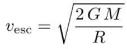Courses

# Test: Kepler’s Laws & Satellites

## 10 Questions MCQ Test Physics Class 11 | Test: Kepler’s Laws & Satellites

Description
This mock test of Test: Kepler’s Laws & Satellites for JEE helps you for every JEE entrance exam. This contains 10 Multiple Choice Questions for JEE Test: Kepler’s Laws & Satellites (mcq) to study with solutions a complete question bank. The solved questions answers in this Test: Kepler’s Laws & Satellites quiz give you a good mix of easy questions and tough questions. JEE students definitely take this Test: Kepler’s Laws & Satellites exercise for a better result in the exam. You can find other Test: Kepler’s Laws & Satellites extra questions, long questions & short questions for JEE on EduRev as well by searching above.
QUESTION: 1

### India’s first artificial satellite was:

Solution:

India's first artificial satellite was Aryabhata. Launched by soviet union on 19  April 1975

QUESTION: 2

Solution:
QUESTION: 3

### Weightlessness is:

Solution:

Weight becomes zero in a freefall or when the total force on your body is zero. For example, in a freely falling lift the contact force on your body is zero, so the weighing​ machine would show zero reading. So you are weightless.

QUESTION: 4

Different planets have different escape velocities because:

Solution:

The formula for calculating the escape velocity from the surface of a celestial body (e.g. a planet) is:where G is the universal gravitation constant, M is the planet’s mass and R is its radius. Different planets have different mass and radius - and therefore different escape velocity.

QUESTION: 5

Kepler’s second law states that the straight line joining the planet to the sun sweeps out equal areas in equal time. The statement is equivalent to saying that:

Solution:

According to the second law the orbital radius and angular velocity of the planet in the elliptical orbit will vary. The planet travels faster when closer to the Sun, then slower when farther from the Sun. Hence we can say that the transverse acceleration is zero while radial and longitudinal accelerations are not zero.

QUESTION: 6

A satellite which appears to be at a fixed position at a definite height to an observer is called:

Solution:

As the relative velocity of the satellite with respect to the earth is zero, it appears stationary from the Earth surface and therefore it is called is geostationary satellite or geosynchronous satellite.

QUESTION: 7

The squares of the periods of revolution of the planets are proportional to the ___________of their semimajor axis of its orbit.

Solution:

The Law of Periods: The square of the period of any planet is proportional to the cube of the semimajor axis of its orbit.

QUESTION: 8

Which is untrue about orbital velocity?

Solution:

1.Orbital velocity of satellite is independent of mass of the satellite
2.It decreases with increase in the radius of the orbit and with increase in the height of the satellite
3.it depends on the mass and radius of the planet about which the satellites revolves.
4.The angular momentum of a satellite of mass m moving with velocity vo in an orbit of radius r=(R+h) is given by L=√GMm2r

QUESTION: 9

A satellite moves in a circular orbit around earth. The radius of this orbit is one half that of moon’s orbit. The satellite completes one revolution in:

Solution:

The time period of revolution of the moon around the earth = 1 lunar month.
► Ts/Tm = (rs/rm)3/2 = (1/2)3/2
►  Ts  = (2)-3/2 lunar month

QUESTION: 10

Kepler’s law of areas can be understood as a consequence of:

Solution:

Conservation of angular momentum:
L= 2m dA/dt